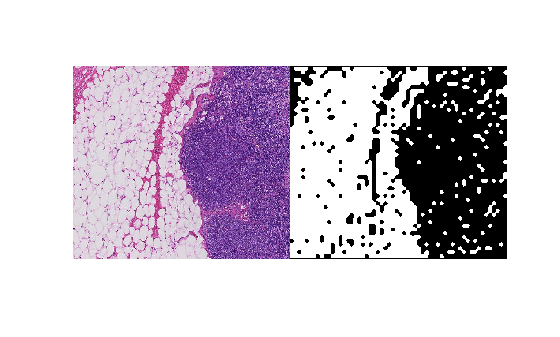# sub2world

Convert pixel subscripts to block subscripts

Since R2021a

## Syntax

``world = sub2world(bim,pixelsub)``
``world = sub2world(bim,pixelsub,Level=L)``

## Description

example

````world = sub2world(bim,pixelsub)` converts the pixel subscripts, `pixelsub`, to the block subscript of the block containing the corresponding pixel. ```
````world = sub2world(bim,pixelsub,Level=L)` additionally specifies the resolution level `L` to use in a multiresolution image.```

## Examples

collapse all

Convert pixel subscripts from one level to another via the world coordinates.

Create a blocked image from a sample image included with the toolbox.

`bim = blockedImage("tumor_091R.tif");`

Create a binary mask at the coarsest resolution level.

`bmask = apply(bim, @(bs)imbinarize(im2gray(bs.Data)),Level=3);`

Define a region of interest (ROI) in the finest resolution level in pixel subscripts.

```pixelStartLevel1 = [1700 1550]; pixelEndLevel1 = [2100 2000]; imagePixelSubs = [pixelStartLevel1; pixelEndLevel1];```

Get the image data from the ROI at the finest resolution level.

```roiImage = getRegion(bim,pixelStartLevel1,pixelEndLevel1,Level=1); size(roiImage)```
```ans = 1×3 401 451 3 ```

Convert the pixel subscripts that define the ROI into world coordinates. By default, `sub2world` converts the coordinates at the finest resolution level.

`worldRegion = sub2world(bim,imagePixelSubs);`

Convert the world coordinates of the ROI to pixel subscripts of the mask.

`maskPixelSubs = world2sub(bmask,worldRegion);`

Get the mask data from the ROI.

```roiMask = getRegion(bmask,maskPixelSubs(1,:),maskPixelSubs(2,:)); size(roiMask)```
```ans = 1×2 51 58 ```

View the region of interest and the corresponding mask.

`montage({roiImage,roiMask})`## Input Arguments

collapse all

Blocked image, specified as a `blockedImage` object.

Pixel subscripts, specified as a K-by-N integer-valued vector, where K is the number of pixels. N is equal to or less than the number of dimensions of the blocked image `bim`. For example, you can exclude a color channel from the conversion by specifying the pixel subscripts with one less dimension than the blocked image.

Resolution level, specified as a positive integer that is less than or equal to the number of resolution levels of `bim`.

## Output Arguments

collapse all

World coordinates, returned as a K-by-N numeric array, where K is the number of pixels and N is the dimensionality of the coordinates.

## Version History

Introduced in R2021a

expand all